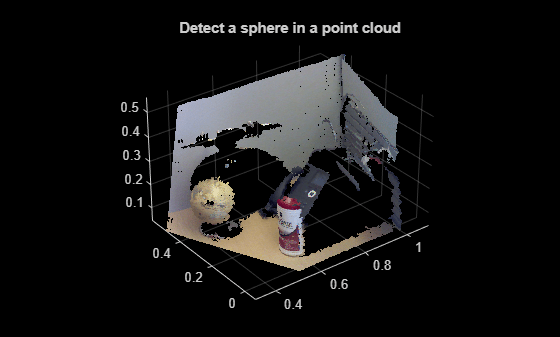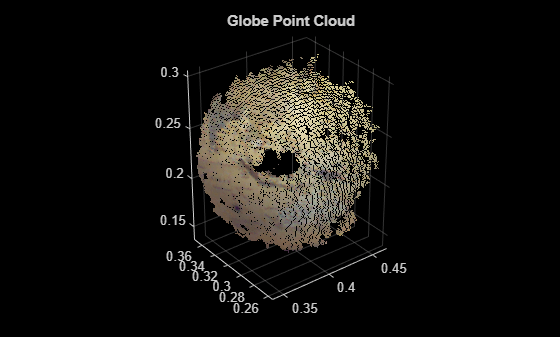# sphereModel

Object for storing a parametric sphere model

## Description

The `sphereModel` object stores a parametric sphere model.

## Creation

### Syntax

``model = sphereModel(params)``

### Description

example

````model = sphereModel(params)` constructs a parametric sphere model from the specified parameters. The `params` argument is a 1-by-4 numeric vector that determines the value of the `Parameters` property.```

## Properties

expand all

Sphere model parameters, stored as a 1-by-4 numeric vector. The four parameters [a b c d] satisfy this equation for a sphere:

`${\left(x-a\right)}^{2}+{\left(y-b\right)}^{2}+{\left(z-c\right)}^{2}={d}^{2}$`

Center of the sphere, stored as a 1-by-3 vector of form [xc yc zc]. The elements xc,yc,zc specify the x-, y-, and z-coordinates of the center coordinates of the sphere, respectively.

Radius of the sphere, stored as a scalar value.

## Object Functions

 `plot` Plot parametric model

## Examples

collapse all

`load("object3d.mat");`

Display point cloud.

```figure pcshow(ptCloud) xlabel("X(m)") ylabel("Y(m)") zlabel("Z(m)") title("Detect a sphere in a point cloud")```Set the maximum point-to-sphere distance (1cm), for sphere fitting.

`maxDistance = 0.01;`

Set the region of interest to constrain the search.

```roi = [-inf,0.5;0.2,0.4;0.1,inf]; sampleIndices = findPointsInROI(ptCloud,roi);```

Detect the globe in the point cloud and extract it.

`model = pcfitsphere(ptCloud,maxDistance,SampleIndices=sampleIndices);`

Plot the sphere.

```hold on plot(model)```## Version History

Introduced in R2015b